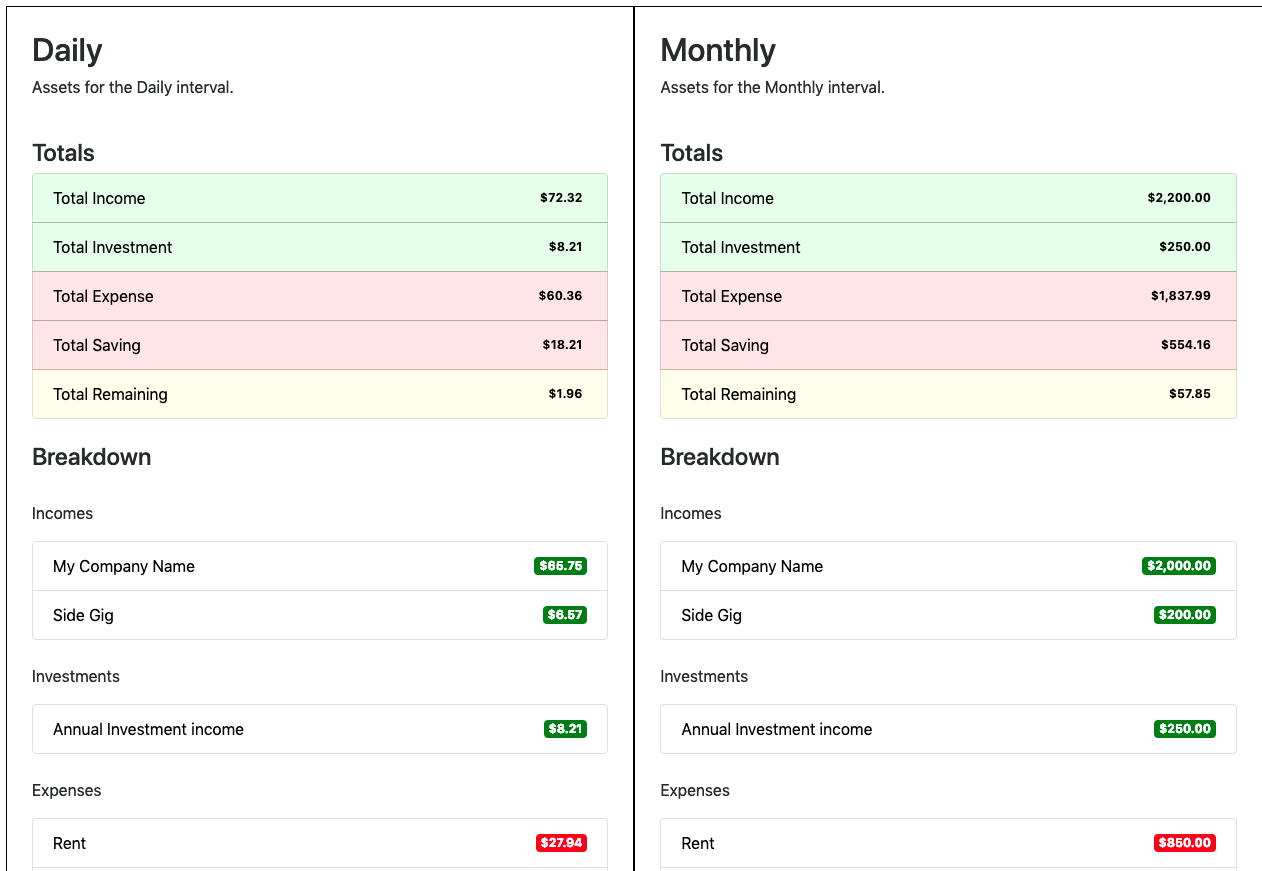Nervous Parrot Muttering

# npm

Have ideas to improve npm?Join in the discussion! »

## asset-tool

1.0.1 • Public • Published

# Asset tool

NOTE: Only USD currently supported

This tool is geared for personal use and is not recommended / robust enough to be used in a production environment.

The workflow for using this tool is as follows:

1. Type out your income and expenses by interval (daily, monthly, annual) in a Node.js script that imports this module.
2. Run the file to calculate your total earnings and expenses per each interval.
3. Save the summary of the calculations to a pdf or html file, or simply print the summary to your console.

See example.js for an example script that uses this tool to calculate a daily, monthly, and annual breakdown of your finances.

The following is an image of the html summary generated from running example.js.## Usage

### Install

`npm i -s asset-tool`

Import the module

`const Asset = require('asset-tool')`

### QuickStart

Simply use example.js as a template, or read on from here to learn how to setup the tool from the ground up.

### Declare your assets

`Asset.construct(amount, type, interval, label)`

• `amount`: A number value in dollars of the amount of the asset.
• Can be an array of numbers that will be averaged. (e.g. `[200, 400]` will be averaged to `300`)
• `type`: Can be a value from `Asset.TYPE` (see Static Values)
• `interval`: Can be a value from `Asset.INT` (see Static Values)
• `label`: A string identifying the asset (e.g. 'Rent')
• `isCents`: defaults to `false`, if set to `true`, amount will be in cents.

Example

Example of average amounts

Example when an `amount` of \$19.99 is specified in cents

### Declare using default constructor

`new Asset(amount, {type: '', interval: '', label: ''}, isCents`

• `amount`: A number value in dollars of the amount of the asset.
• Can be an array of numbers that will be averaged. (e.g. `[200, 400]` will be averaged to `300`)
• Always Required
• `type`: Can be a value from `Asset.TYPE` (see Types of Assets)
• Optional. Defaults to `Asset.TYPE.EXPENSE`
• `interval`: Can be a value from `Asset.INT` (see Available Intervals)
• Optional. Defaults to `Asset.INT.MONTHLY`
• `label`: A string identifying the asset (e.g. 'Rent')
• Optional. Defaults to `Unlabelled`
• `isCents`: defaults to `false`, if set to `true`, amount will be in cents.
• Optional. Defaults to `false` (dollars)

### Include an Asset in calculations

`include()` each asset that you want to participate in final calculations. If you create assets and manipulate them with calculations, then you only want to `include()` the final asset.

Example:

### View results

You can run calculations and view the breakdown of all on your `include()`'d assets from:

1. The command line.
2. A generated HTML or PDF file.

To generate an html or pdf file at the end of your script include:

See Generate Summary for an overview of generating a pdf or html.

To print your totals for each interval (daily, monthly, annual) in the command line, at the end of your script include:

To print your totals and the breakdown for a specific interval, at the end of your script include:

## Generate Summary

A `summary` contains your totals by interval and the breakdowns that compose those totals.

By default, `Asset.generateSummary()` will generate an HTML file called `summary.html` in the directory that the script is run.

### PDF options

To generate a pdf you have the following options. The example shows the defaults, the comments show possible values.

### HTML options

Additionally, you can specify the html file's path.

### Shared options

For both html and pdf summaries, the following options are available.

## Types of Assets

An asset can be one of the following types,

1. `income`: Amount gained from employment(s) (net positive)
2. `investment`: Amount gained from investment(s) (net positive)
3. `saving`: Amount saved (net negative)
4. `expense`: Amount spent (net negative)

and can programmatically be accessed using the following static properties:

e.g. `Asset.TYPE.INCOME`.

Types are currently only used for grouping in the `summary`'s financial breakdown.

Future plans will allow types to have properties attached to them, for instance,

• A `saving` could accept a percent return for an interval.
• An `investment` could accept the amount invested (net negative) and projected rates of return (net positive0).
• An `income` could accept total tax percentages.

## Available Intervals

An interval can be one of the following,

1. `daily`
2. `monthly`
3. `annual`

and can programmatically be accessed using the following static properties:

e.g. `Asset.INT.DAILY`.

## TODO

• Add modifiers to certain types as specified in Types of Assets
• Add tests and CI script to run those tests
• Add quarterly intervals?
• Make more robust / less hacky

## Keywords

### Install

`npm i asset-tool`

0

1.0.1

ISC

458 kB

11

### Homepage

github.com/Ebonsignori/asset-tool#readme

### Repository

github.com/Ebonsignori/asset-tool